Aswin Vijayakumar — Published On October 7, 2020 and Last Modified On October 7th, 2020

This article was published as a part of the Data Science Blogathon.

Values offer Focus amidst the Chaos” – Glenn C. Stewart

## Introduction

Joseph Fourier born in 1768 discovered that a planet with the size of Earth and equivalent distance from the Sun is meant to have lower temperatures than what is measured on the earth’s surface. His inference was that the Earth is warmed by the incoming solar radiation. Joseph Fourier postulated that heat flow is proportional to an infinitesimal difference in temperature between adjacent molecules.

Out of my interest in understanding heat flow and circulation of air within a building with circulation realms as windows, I did a study on spatial data containing a room with 3 access areas. Fascinating! just by using my notebook, I was able to conclude some exciting results on Lagrangian Value Functions, the responsibility to circulate and displace air.

I used a Lagrangian Value Function to construct a state space that used two exponential functions — one with a negative variable (i.e. exp^(x)) and the other with a positive variable (i.e. exp^(-x)). I assumed a Poisson distribution considering collisions of random particles arranged in the room space as the behavioral model for constructing a state-space representing distance as the characteristic variable.

I called this retention of some object within the space. Retention is either not too far and not too close from the aspects of circulation realms.

This implies that the distance will be the deciding factor relevant to the deterioration or increase of a hypothetical value function such as characteristic circulation or heat flow.

The things I observed are novel, I learned about three new statistics — LM, Chi-squared and F-test.

I applied a Lagrangian Value Function based on Machine Learning with inverse exponentials termed as affinity indicating not too close and the regular exponentials termed as distance indicating not too far.

When I transformed the Lagrangian State Space into a Characteristic Loss Function using Complex Analysis, I was able to visualize chi-squared distribution on one aspect of the circulation realms, the F-statistic on the second aspect, and a Mahalanobis distribution for the main aspect. To rephrase the problem, when I applied a heteroskedastic LM (Lagrangian Multiplier) statistic on spatial data, I obtained Fourier Transformed frequency domains as F-distribution, Chi-Squared distribution, and a Gaussian-like distribution for a window, a door, and another large window respectively. I learned that there are these three distributions that help us design the placement of windows and doors to form the circulation realms within a room of the building.

Moreover, I was able to verify the findings from a book on Econometrics as well as a book on Elements of Statistical Learning that heteroskedasticity works against the Gauss-Markov problem.

#### *Fact

Person 1: What is the difference between cascade relationship vs stage relationship?

Person 2: Cascading is about taken place when the process is incomplete, but the stage happens when the process is complete and then the next one is going to start

## What is Boxing and Unboxing in Computer Language?

Example

``````int i = 123;      // a value type
object o = i;     // boxing
int j = (int)o;   // unboxing``````

` `

MDSN Definition

Boxing is used to store value types in the garbage-collected heap. Boxing is an implicit conversion of a value type to the type `object` or to any interface type implemented by this value type.

Unboxing is an explicit conversion from the type `object` to a value type or from an interface type to a value type that implements the interface.

## What is F-unboxing?

Boxing and Unboxing of a DEA problem start with Data Management Units, especially the input /output Data Management Units (DMUs). F-distribution is a comparison of the errors of two populations. In a  DEA problem, this happens when a pseudo-unit-weighted regression is performed using Output DMUs, through Input Data/DMU, and then finally Output Data. this is a three step process of unboxing.

## What is Chi-unboxing?

The chi-Squared distribution is a goodness of fit of an observed distribution to the theoretical one. In our case, it is quite similar to comparing the Input DMUs and Input Data. In chi-squared, the exposures of data are qualitatively analyzed and kept as a normal distribution.

## What is Mahalanobis-unboxing?

Drawing the Output DMUs from a Mahalanobis Distribution, a T-Test is conducted on the two distributions – the Input Data and the Output Data.

## Exposures in Linear Regression

Exposure is the coefficient of a variable used in the regression. Consider the Data Envelopment Analysis (DEA) problem as the ratio of weighted sums, explained here:

In heteroskedastic LM statistic, the residual sum of squares (RSS) is the exposure to the exposure of a variable. This makes F-statistic and chi-squared to be technically substituted by the LM statistic because both use the residual sum of squares. F-statistic is significantly closer to imagining a hypothesis derived from the LM-statistic, whereas the chi-squared is relatively dependent on the R2 score performed on true and predicted values.

#### *Joke

Person 1: Do you think Hessian which is grad square is political in nature?

Person 2: Hessian is a search vector to several parametric variables; hence, it is political in nature.

Using constrained optimization, the found distributions are representable using Minimum Mean Squared Estimation (MMSE). MMSE as per Stanford Winter presentation on Statistics and Confidence Intervals has been provided here. As MMSE can be plugged into the DEA model as the weighted output, with the weighted input being a p-value, it is suited for application towards transforming the model to these three distributions.

## How were the statistical tests conducted?

The F-statistic, chi-squared and the Mahalanobis considered input vector as a normal distribution, output data a uniform distribution and the input DMUs as coming from a Gaussian.

The formulation of output DMU (s) are changed based on desired use cases.

The F-distribution considers Spearman rank correlation as input DMU (s) to conclude the results whereas the chi-squared distribution considers an MMSE term to conclude.

The Mahalanobis condition considers the Mahalanobis distance vector as input DMU (s) in order to conclude its result with the other distributions.

## Object Constraint Language (OCL)

…A declarative language describing rules applying to Unified Modeling Language (UML) models…

(1) Object constraint language (OCL) is defined to impose constraints on the structural model.

(2) These include variable assignments, for loops, sub-collection extraction from a base collection, etc. A collection is either a Set, Sequence or Bag.

(3) The objects inside can have a user-defined type and attributes similar to OOP style programming.

OCL(s) describe the preconditions and postconditions of a single operation in terms of Boolean representation. In this article, I use OCL(s) to represent a function transformation from MMSE or Gaussians to the selected distributions.

Given below are statistical distributions obtained from a DEA (Data Envelopment Analysis) Model.

## Using a DEA Model from Spearman Rank Correlation

F-unboxing is defined as obtaining the output weights of uniform distribution such that the output DMU (s) use Spearman R correlation.

The output weights obtained from the DEA Model using Spearman R correlation as DMU(s).

This implies when you unbox a DEA Model from Spearman R correlation, the first unboxing results in the Input Weights and the second unboxing results in the F-distribution provided Output Data is Uniform Distribution and Input is Normal vector.

(1) F-Test conducted for an F-unboxing,

(2) Chi-Squared Test conducted for an F-unboxing,

(3) T-Test conducted for a F-unboxing## Using a DEA Model from MMSE

Chi-unboxing is defined as obtaining the output weights of uniform distribution by using MMSE as DMU (s) and evaluating the input vector and input DMU (s) together for the Chi-Squared test.

The output weights obtained from an MMSE model using Gaussian Vectors as Inputs and MMSE from Uniform Distributions as DMU(s).

This implies when you unbox a DEA Model from MMSE, the first unboxing results in Input DMU (s) which is used to evaluate the Chi-Squared Distribution Tests.

(1) F-Test conducted for a Chi-unboxing, (2) Chi-Squared Test conducted for a Chi-unboxing, (3) T-Test conducted for a Chi-unboxing

## Using a DEA Model from MMSE by taking Mahalanobis

Mahalanobis-unboxing is defined as obtaining the output weights of uniform distribution by using Mahalanobis Distance as DMU (s) and evaluating the output for T-Test.

The input weights obtained from a Mahalanobis model using Gaussian Vectors as Inputs and Mahalanobis from Uniform Distributions as DMU(s).

This implies when you unbox a DEA Model from the Mahalanobis Distance vector, the first unboxing results in Input Weights and the second unboxing results in Mahalanobis-unboxing.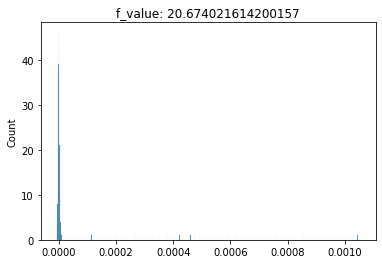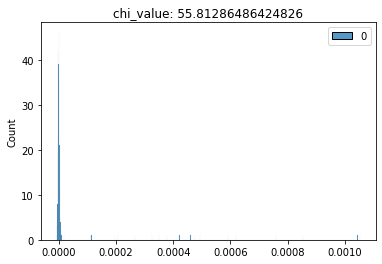(1) F-Test conducted for a Mahalanobis-unboxing, (2) Chi-Squared Test conducted for a Mahalanobis-unboxing, (3) T-Test conducted for a Mahalanobis-unboxing

## Reflection on the Findings

From each distribution: (1), (2) and (3):(1) Consider these statistical distributions represent the frequency of any activity, in such cases:

(a) These distributions can be considered as the Frequency Domain generated from a Custom Metric which is relevant to the Lagrangian Value Function

(b) Such a Frequency Domain is obtained from Fourier Analysis or Fourier Transform

(2) The results from the three domains are not bad for Mahalanobis and F-test. For a Chi-test, the p-values are very small indicating that the observed and expected vectors are very close to each other.

(3) This implies FFT transforms will be highly significant for visualizing Chi-Squared Distributions.

(4) We can use DEA Models for representing the F-distribution and the Mahalanobis distribution.

(5) We found two methods that can produce a spectrum of randomly generated chi-squared and F or Mahalanobis distributions.

(6) Such spectrums are useful for visualizing the input parameter, output parameter, and the intermediary variables that represent the state space of the system.

## Visualizations

When I visualized the L2 Norm of weights of the output frequency of these three distributions, I found that:

(1) F-distribution frequency requires average values for weights among Chi and Mahalanobis.

(2) Chi-squared distribution frequency requires the greatest values for weights.

(3) Mahalanobis distribution frequency requires least values for weights.

This indicates the FFT transform results obtained based on the experiment and the DEA results with correct operations do show some level of concordance. Shown are heatmaps of the three distributions: — When input weights constraints are ≥ 0About the Images below: (1) Heatmap of F-unboxing, (2) Heatmap of Chi-Squared unboxing, and (3) Heatmap of Mahalanobis unboxing frequencies with Output Weights of DEA Model as 2-dimensional Matrix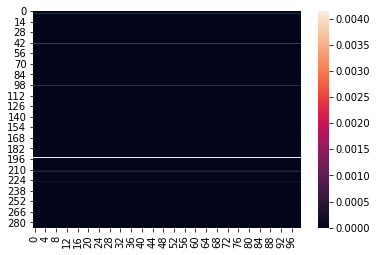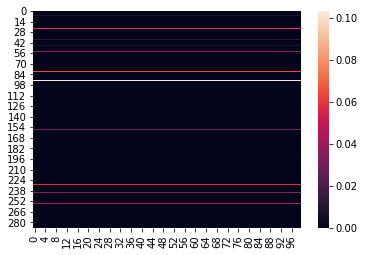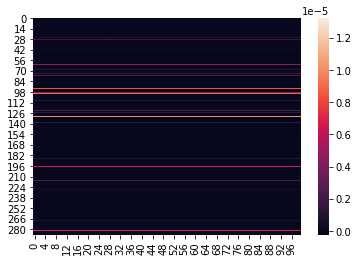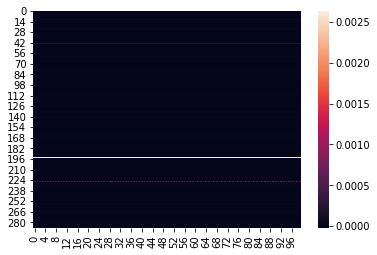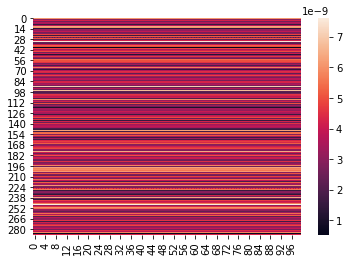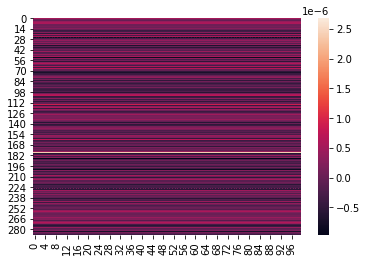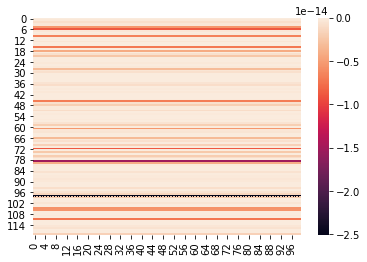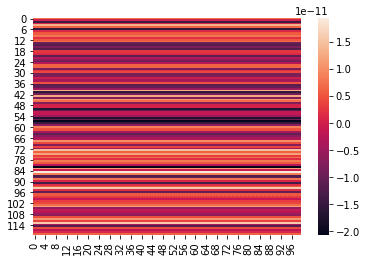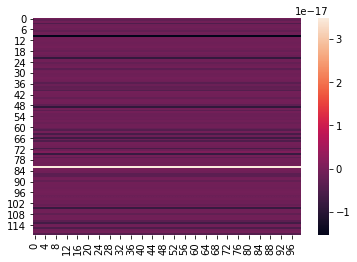*Joke

Person 1: There is a joke that Chi-squared distribution is Gaussian.

Person 2: Chi-squared is probabilistic on outlier theory as well as exhibits statistical concordance. It is an elegantly defined statistical distribution.

*Joke

Person 1: There is a joke that second-order derivatives are useful to none, is that true or false?

Person 2: No, acceleration is a second-order derivative of position, and it is a useful metric.

If we define a boxing and unboxing technique that relies on Data Envelopment Analysis, it is my best assumption that these representations become useful. The visualizations are purely for imagining the state-space represented by our distributions.

Each chosen distribution and the Gaussian input vector represent the material properties.

Such boxing and unboxing operations are useful for Use case modeling.

Appendix – Boxing Operations vs Unboxing Operations (OCL-s)

— A 2D Matrix of Output weights is used to evaluate the distributions, whereas a 1D Vector of Output weights is used to evaluate the statistical tests.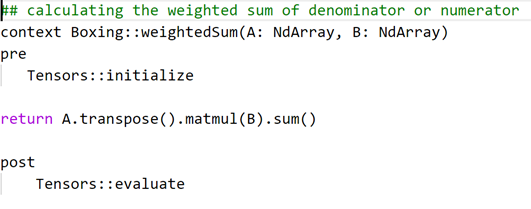Here is an example of OCL operation defined for weightedSum

— The Input Data is drawn from Normal Distribution whereas the Output Data which is mapped from Input Data is drawn from Uniform Distributions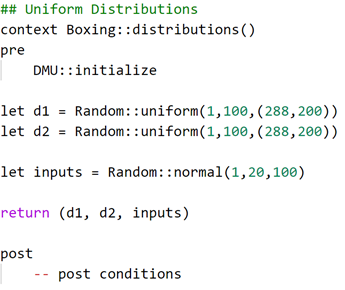This is an example of boxing distributions used to manage inputs

— DMU(s) are initialized with 3 different distributions in order to produce results in boxing and unboxing. Spearmann R has been selected for F, MMSE has been selected for Chi-Squared and Mahalanobis has been selected for Mahalanobis.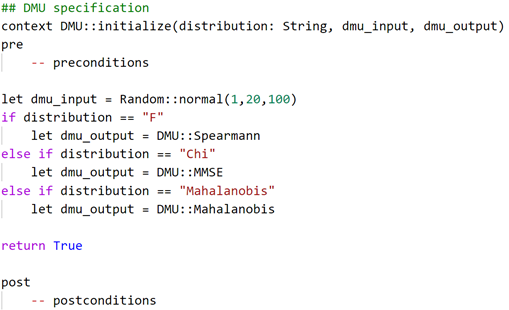The DMU(s) get initialized from F, Chi, and Mahalanobis distributions into Spearman, MMSE, and Mahalanobis respectively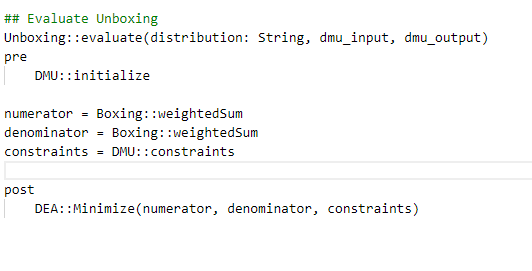DEA Minimization is used.